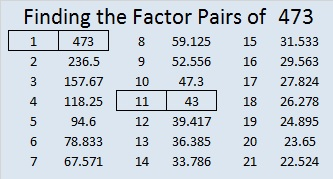# Just a Little 473 Cake

Today is our wedding anniversary. Today I tried to spend my time with my husband instead of the computer. Hence this is a very short and plain post.

The middle digit of 473 equals the sum of the other two digits which means that 473 can be evenly divided by 11.

Five years from now I could make one of these cakes for our anniversary!473 is the sum of some consecutive prime numbers two different ways. See if you can find them yourself. Then check the comments to see if you were right.

• 473 is a composite number.
• Prime factorization: 473 = 11 x 43
• The exponents in the prime factorization are 1 and 1. Adding one to each and multiplying we get (1 + 1)(1 + 1) = 2 x 2 = 4. Therefore 473 has exactly 4 factors.
• Factors of 473: 1, 11, 43, 473
• Factor pairs: 473 = 1 x 473 or 11 x 43
• 473 has no square factors that allow its square root to be simplified. √473 ≈ 21.74856